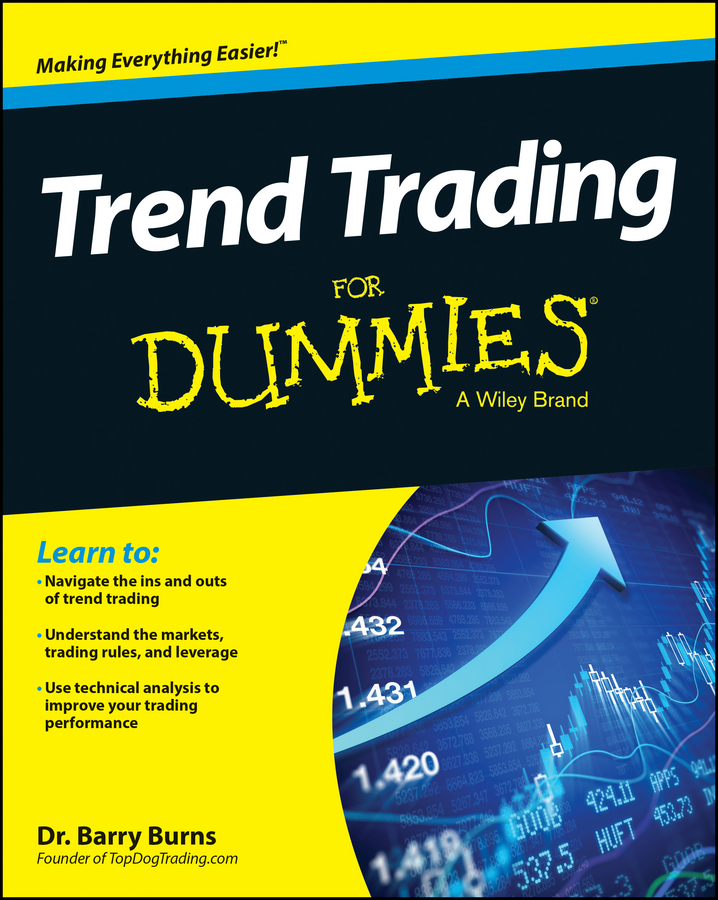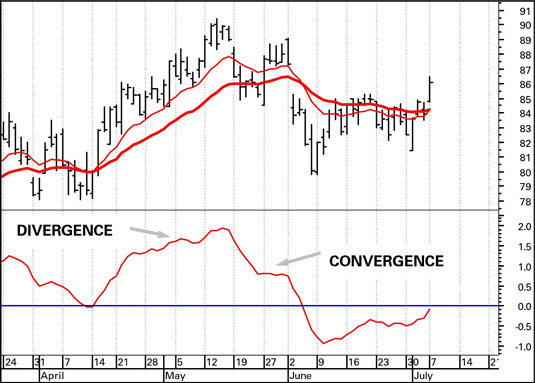If you look at any two moving average crossovers in a trading chart, you see that at a turning point, the short moving average converges to the price and the long-term moving average converges, a bit later, to the short-term one. Similarly, after a crossover, the two moving averages diverge from one another.

Here are the convergence and divergence basics you need to know:

• Convergence: When two moving averages converge, the trend may be coming to an end. Convergence is therefore an early warning. Because moving averages are always lagging indicators, measuring convergence is a way of anticipating a crossover.

At a peak, one way to look at the convergence is to say that short-term demand is faltering — traders are failing to produce new higher closes. The trend is still in place, as shown by the long-term moving average. At a price bottom, you can interpret the short-term moving average falling at a lesser pace as selling interest (supply) falters.

• Divergence: Conversely, when you can see a lot of daylight between two moving averages, they’re diverging, and that means the trend is safe from a crossover, at least for another few periods. In practice, abnormally wide divergence tends not to be sustainable and can be a warning of prices having reached an extreme ahead of reversing.

To calculate convergence and divergence, you simply subtract the long-term moving average from the short-term one. This figure shows a 12-day and 26-day moving average in the top window. In the bottom window is the result of subtracting the 26-day moving average from the 12-day moving average, which is the convergence-divergence indicator:• When the indicator line is rising, the two averages are diverging.

• When the line is falling, the averages are converging.

• At zero difference between the two averages, you have the crossover. You can verify this crossover by checking the actual moving averages on the price chart.